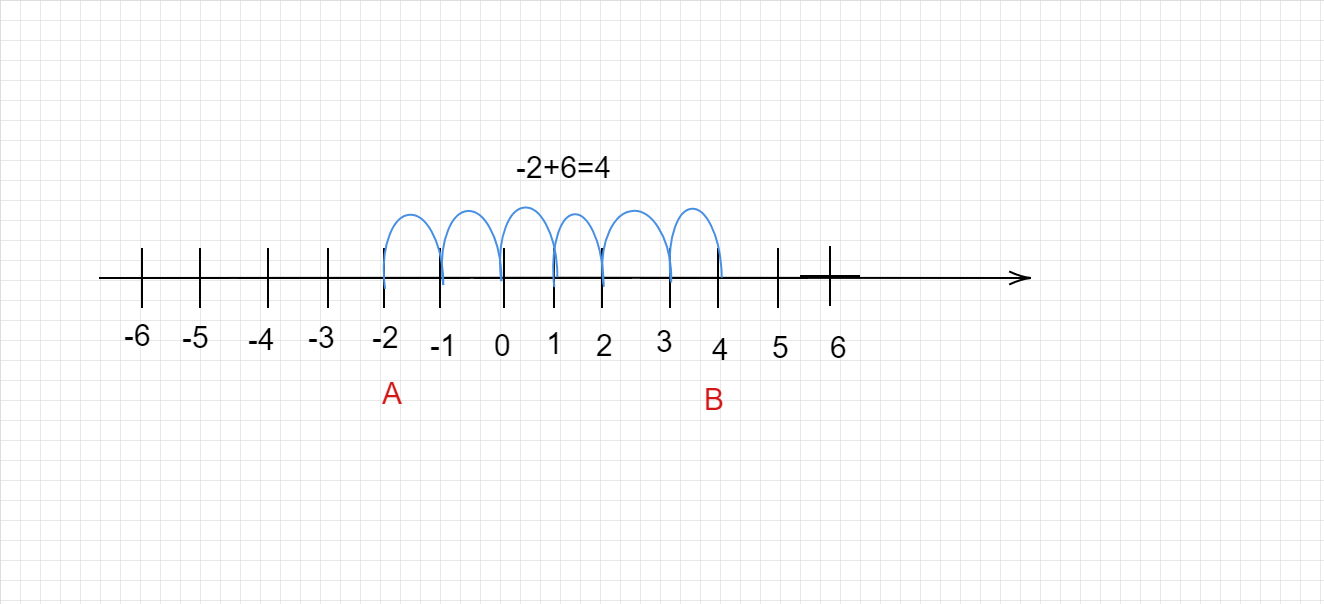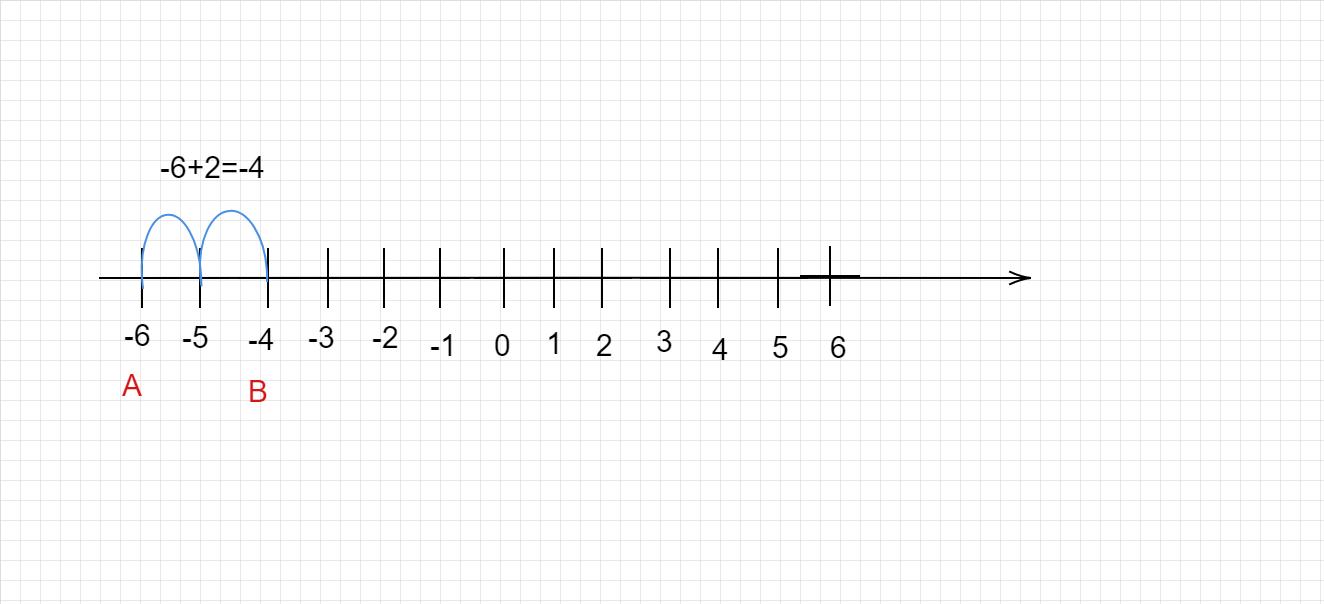# Find the solution of the following additions using a number line:(a) $(-2) + 6$ (b) $(-6)+2$

Given :

The given terms are,

(a) $(-2) + 6$                  (b) $(-6)+2$.

To do :

We have to find the solution of the given additions using the number line.

Solution :

In order to add two integers on a number line, we follow the following steps:

STEP I: Draw a number line and mark integers on it.

STEP-II: Start from the point representing the first number on the number line.

STEP III: Move as many units as the second number to the

(i) right of the first number, if the second number is positive.

(ii) left of the first number, if the second number is negative.

STEP IV: Obtain the number representing the point reached in the previous step.

This number represents the required sum of the given integers.

(a) Start from point A and move 6 units to the right. B(4) is the required point which represents $(-2)+6$.(b) Start from point A and move 2 units to the right. B(-4) is the required point which represents $(-6)+2$.Updated on: 10-Oct-2022

24 Views